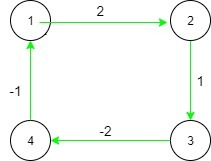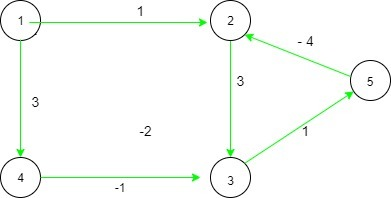# Check whether the cost of going from any node to any other node via all possible paths is same

Given adjacency list representation of a directed graph, the task is to check whether the cost of going from any vertex to any other vertex via all possible paths is equal or not. If there is a cost c for going from vertex A to vertex B, then the cost to travel from vertex B to vertex A will be -c.

Examples:

Input: arr[][] = {{0, 2, 0, 1}, {-2, 0, 1, 0}, {0, -1, 0, -2}, {-1, 0, 2, 0}}
Output: Yes
Explanation:Here the cost of going from any node to any other node is equal for all possible paths. For example, if we go from 1 to 4 via (1 -> 2 -> 3 -> 4) whose cost is (2 + 1 + (-2)) i.e. 1 and via ( 1 -> 4 ) which is a reverse edge whose cost is 1. Similarly, the cost for all other paths is equal.

Input: arr[][] = {{0, 1, 0, 3, 0}, {-1, 0, 3, 0, 4}, {0, -3, 0, 1, 1}, {-3, 0, -1, 0, 0}, {0, -4, -1, 0, 0}}
Output: No
Explanation:For the following two paths from edge 1 to 4, (1 -> 2 -> 3 -> 4), Cost = (1 + 3 + 1) = 5 and (1 -> 4), Cost = 3. Since the costs are difference, the answer is No

## Recommended: Please try your approach on {IDE} first, before moving on to the solution.

Approach: The idea is to maintain two arrays dis[] which maintains the distance of the paths travelled and visited[] which maintains the visited vertices. The graph is stored using 2D vector of pairs. The first value of the pair is the destination node and the second value is the cost associated with it. Now, DFS is run on the graph. The following two conditions occur for every vertex:

1. If the next node to reach is not visited, then the dis array is updated with the value dis[current_node] + cost of the new edge found from the 2D vector i.e. current node to next node to reach and the same function is called with the unvisited same node.
2. If the node is visited, then the distance of the next node to reach is compared with the dis[curr] + cost of edge to reach the next node. If they are equal, then the boolean variable flag is updated with true and the loop is continued for the next vertices.

Below is the implementation of the above approach:

## CPP

 `// C++ implementation of the above approach ` `#include ` `using` `namespace` `std; ` `vector > adj; ` ` `  `// Initialize distance and visited array ` `int` `vis = { 0 }, ` `    ``dist = { 0 }, ` `    ``flg; ` ` `  `// Function to perform dfs and check ` `// For a given vertex If the distance ` `// for all the paths is equal or not ` `void` `dfs(``int` `curr) ` `{ ` `    ``vis[curr] = 1; ` `    ``for` `(``int` `i = 0; i < adj[curr].size(); i++) { ` ` `  `        ``// Checking the next node to reach ` `        ``// is visited or not ` `        ``if` `(vis[adj[curr][i].first]) { ` ` `  `            ``// Case 2: comparing the distance ` `            ``if` `(dist[adj[curr][i].first] ` `                ``!= dist[curr] + adj[curr][i].second) ` `                ``flg = 1; ` `        ``} ` `        ``else` `{ ` ` `  `            ``// Case 1: Adding the distance ` `            ``// and updating the array ` `            ``dist[adj[curr][i].first] = dist[curr] ` `                                       ``+ adj[curr][i].second; ` ` `  `            ``// Calling the function again with the ` `            ``// same node ` `            ``dfs(adj[curr][i].first); ` `        ``} ` `    ``} ` `} ` ` `  `// Driver code ` `int` `main() ` `{ ` `    ``int` `n = 4, m = 4; ` `    ``flg = 0; ` `    ``// Creating the graph as mentioned ` `    ``// in example 1 ` `    ``adj.push_back({ 1, 2 }); ` `    ``adj.push_back({ 0, -2 }); ` `    ``adj.push_back({ 2, 1 }); ` `    ``adj.push_back({ 1, -1 }); ` `    ``adj.push_back({ 3, -2 }); ` `    ``adj.push_back({ 2, 2 }); ` `    ``adj.push_back({ 0, -1 }); ` `    ``adj.push_back({ 3, 1 }); ` `    ``for` `(``int` `i = 0; i < n; i++) { ` `        ``if` `(flg) ` ` `  `            ``// If for any vertex, flg is true, ` `            ``// then the distance is not equal ` `            ``break``; ` ` `  `        ``if` `(!vis[i]) ` ` `  `            ``// Calling the DFS function if ` `            ``// the vertex is not visited ` `            ``dfs(i); ` `    ``} ` `    ``if` `(flg) ` `        ``cout << ``"No"` `<< endl; ` `    ``else` `        ``cout << ``"Yes"` `<< endl; ` `    ``return` `0; ` `} `

## Java

 `// Java implementation of the above approach ` `import` `java.util.*; ` ` `  `class` `GFG { ` `    ``static` `class` `pair { ` `        ``int` `first, second; ` ` `  `        ``public` `pair(``int` `first, ``int` `second) { ` `            ``this``.first = first; ` `            ``this``.second = second; ` `        ``} ` `    ``} ` ` `  `    ``static` `Vector[] adj = ``new` `Vector[``100005``]; ` ` `  `    ``// Initialize distance and visited array ` `    ``static` `int``[] vis = ``new` `int``[``100005``]; ` `    ``static` `int``[] dist = ``new` `int``[``100005``]; ` `    ``static` `int` `flg; ` ` `  `    ``// Function to perform dfs and check ` `    ``// For a given vertex If the distance ` `    ``// for all the paths is equal or not ` `    ``static` `void` `dfs(``int` `curr) { ` `        ``vis[curr] = ``1``; ` `        ``for` `(``int` `i = ``0``; i < adj[curr].size(); i++) { ` ` `  `            ``// Checking the next node to reach ` `            ``// is visited or not ` `            ``if` `(vis[adj[curr].get(i).first] > ``0``) { ` ` `  `                ``// Case 2: comparing the distance ` `                ``if` `(dist[adj[curr].get(i).first] !=  ` `                    ``dist[curr] + adj[curr].get(i).second) ` `                    ``flg = ``1``; ` `            ``} ``else` `{ ` ` `  `                ``// Case 1: Adding the distance ` `                ``// and updating the array ` `                ``dist[adj[curr].get(i).first] =  ` `                    ``dist[curr] + adj[curr].get(i).second; ` ` `  `                ``// Calling the function again with the ` `                ``// same node ` `                ``dfs(adj[curr].get(i).first); ` `            ``} ` `        ``} ` `    ``} ` ` `  `    ``// Driver code ` `    ``public` `static` `void` `main(String[] args) { ` `        ``int` `n = ``4``, m = ``4``; ` `        ``flg = ``0``; ` `        ``for` `(``int` `i = ``0``; i < adj.length; i++) { ` `            ``adj[i] = ``new` `Vector(); ` `        ``} ` `        ``// Creating the graph as mentioned ` `        ``// in example 1 ` `        ``adj[``0``].add(``new` `pair(``1``, ``2``)); ` `        ``adj[``1``].add(``new` `pair(``0``, -``2``)); ` `        ``adj[``1``].add(``new` `pair(``2``, ``1``)); ` `        ``adj[``2``].add(``new` `pair(``1``, -``1``)); ` `        ``adj[``2``].add(``new` `pair(``3``, -``2``)); ` `        ``adj[``3``].add(``new` `pair(``2``, ``2``)); ` `        ``adj[``3``].add(``new` `pair(``0``, -``1``)); ` `        ``adj[``0``].add(``new` `pair(``3``, ``1``)); ` `        ``for` `(``int` `i = ``0``; i < n; i++) { ` `            ``if` `(flg == ``1``) ` ` `  `                ``// If for any vertex, flg is true, ` `                ``// then the distance is not equal ` `                ``break``; ` ` `  `            ``if` `(vis[i] != ``1``) ` ` `  `                ``// Calling the DFS function if ` `                ``// the vertex is not visited ` `                ``dfs(i); ` `        ``} ` `        ``if` `(flg == ``1``) ` `            ``System.out.print(``"No"` `+ ``"\n"``); ` `        ``else` `            ``System.out.print(``"Yes"` `+ ``"\n"``); ` `    ``} ` `} ` ` `  `// This code is contributed by PrinciRaj1992 `

## Python3

 `# Python3 implementation of the above approach ` `adj ``=` `[[] ``for` `i ``in` `range``(``100005``)] ` ` `  `# Initialize distance and visited array ` `vis ``=` `[``0``]``*``100005` `dist ``=` `[``0``]``*``100005` `flg ``=` `0` ` `  `# Function to perform dfs and check ` `# For a given vertex If the distance ` `# for all the paths is equal or not ` `def` `dfs(curr): ` `    ``vis[curr] ``=` `1` `    ``for` `i ``in` `range``(``len``(adj[curr])): ` ` `  `        ``# Checking the next node to reach ` `        ``# is visited or not ` `        ``if` `(vis[adj[curr][i][``0``]]): ` ` `  `            ``# Case 2: comparing the distance ` `            ``if` `(dist[adj[curr][i][``0``]] ` `                ``!``=` `dist[curr] ``+` `adj[curr][i][``1``]): ` `                ``flg ``=` `1` `        ``else``: ` ` `  `            ``# Case 1: Adding the distance ` `            ``# and updating the array ` `            ``dist[adj[curr][i][``0``]] ``=` `dist[curr]``+` `adj[curr][i][``1``] ` ` `  `            ``# Calling the function again with the ` `            ``# same node ` `            ``dfs(adj[curr][i][``0``]) ` ` `  `# Driver code ` ` `  `n ``=` `4` `m ``=` `4` `flg ``=` `0` ` `  `# Creating the graph as mentioned ` `# in example 1 ` `adj[``0``].append([``1``, ``2``]) ` `adj[``1``].append([``0``, ``-``2``]) ` `adj[``1``].append([``2``, ``1``]) ` `adj[``2``].append([``1``, ``-``1``]) ` `adj[``2``].append([``3``, ``-``2``]) ` `adj[``3``].append([``2``, ``2``]) ` `adj[``3``].append([``0``, ``-``1``]) ` `adj[``0``].append([``3``, ``1``]) ` ` `  `for` `i ``in` `range``(n): ` `    ``if` `(flg): ` ` `  `        ``# If for any vertex, flg is true, ` `        ``# then the distance is not equal ` `        ``break` ` `  `    ``if` `(vis[i] ``=``=` `0``): ` ` `  `        ``# Calling the DFS function if ` `        ``# the vertex is not visited ` `        ``dfs(i) ` ` `  `if` `(flg): ` `    ``print``(``"No"``) ` `else``: ` `    ``print``(``"Yes"``) ` `     `  `# This code is contributed by mohit kumar 29 `

## C#

 `// C# implementation of the above approach ` `using` `System; ` `using` `System.Collections.Generic; ` ` `  `class` `GFG { ` `    ``class` `pair { ` `        ``public` `int` `first, second; ` `        ``public` `pair(``int` `first, ``int` `second) { ` `            ``this``.first = first; ` `            ``this``.second = second; ` `        ``} ` `    ``} ` `  `  `    ``static` `List[] adj = ``new` `List; ` `  `  `    ``// Initialize distance and visited array ` `    ``static` `int``[] vis = ``new` `int``; ` `    ``static` `int``[] dist = ``new` `int``; ` `    ``static` `int` `flg; ` `  `  `    ``// Function to perform dfs and check ` `    ``// For a given vertex If the distance ` `    ``// for all the paths is equal or not ` `    ``static` `void` `dfs(``int` `curr) { ` `        ``vis[curr] = 1; ` `        ``for` `(``int` `i = 0; i < adj[curr].Count; i++) { ` `  `  `            ``// Checking the next node to reach ` `            ``// is visited or not ` `            ``if` `(vis[adj[curr][i].first] > 0) { ` `  `  `                ``// Case 2: comparing the distance ` `                ``if` `(dist[adj[curr][i].first] !=  ` `                    ``dist[curr] + adj[curr][i].second) ` `                    ``flg = 1; ` `            ``} ``else` `{ ` `  `  `                ``// Case 1: Adding the distance ` `                ``// and updating the array ` `                ``dist[adj[curr][i].first] =  ` `                    ``dist[curr] + adj[curr][i].second; ` `  `  `                ``// Calling the function again with the ` `                ``// same node ` `                ``dfs(adj[curr][i].first); ` `            ``} ` `        ``} ` `    ``} ` `  `  `    ``// Driver code ` `    ``public` `static` `void` `Main(String[] args) { ` `        ``int` `n = 4; ` `        ``flg = 0; ` `        ``for` `(``int` `i = 0; i < adj.Length; i++) { ` `            ``adj[i] = ``new` `List(); ` `        ``} ` `        ``// Creating the graph as mentioned ` `        ``// in example 1 ` `        ``adj.Add(``new` `pair(1, 2)); ` `        ``adj.Add(``new` `pair(0, -2)); ` `        ``adj.Add(``new` `pair(2, 1)); ` `        ``adj.Add(``new` `pair(1, -1)); ` `        ``adj.Add(``new` `pair(3, -2)); ` `        ``adj.Add(``new` `pair(2, 2)); ` `        ``adj.Add(``new` `pair(0, -1)); ` `        ``adj.Add(``new` `pair(3, 1)); ` `        ``for` `(``int` `i = 0; i < n; i++) { ` `            ``if` `(flg == 1) ` `  `  `                ``// If for any vertex, flg is true, ` `                ``// then the distance is not equal ` `                ``break``; ` `  `  `            ``if` `(vis[i] != 1) ` `  `  `                ``// Calling the DFS function if ` `                ``// the vertex is not visited ` `                ``dfs(i); ` `        ``} ` `        ``if` `(flg == 1) ` `            ``Console.Write(``"No"` `+ ``"\n"``); ` `        ``else` `            ``Console.Write(``"Yes"` `+ ``"\n"``); ` `    ``} ` `} ` ` `  `// This code is contributed by 29AjayKumar `

Output:

```Yes
```

Time Complexity: O(V + E) where V is no of vertices and E is no of edges

GeeksforGeeks has prepared a complete interview preparation course with premium videos, theory, practice problems, TA support and many more features. Please refer Placement 100 for details

My Personal Notes arrow_drop_upCheck out this Author's contributed articles.

If you like GeeksforGeeks and would like to contribute, you can also write an article using contribute.geeksforgeeks.org or mail your article to contribute@geeksforgeeks.org. See your article appearing on the GeeksforGeeks main page and help other Geeks.

Please Improve this article if you find anything incorrect by clicking on the "Improve Article" button below.

Article Tags :
Practice Tags :

Be the First to upvote.

Please write to us at contribute@geeksforgeeks.org to report any issue with the above content.